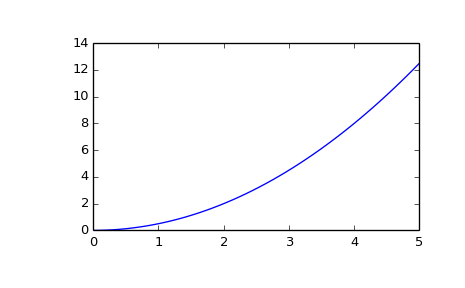# scipy.signal.lsim¶

scipy.signal.lsim(system, U, T, X0=None, interp=True)[source]

Simulate output of a continuous-time linear system.

Parameters: system : an instance of the LTI class or a tuple describing the system. The following gives the number of elements in the tuple and the interpretation: 2: (num, den) 3: (zeros, poles, gain) 4: (A, B, C, D) U : array_like An input array describing the input at each time T (interpolation is assumed between given times). If there are multiple inputs, then each column of the rank-2 array represents an input. If U = 0 or None, a zero input is used. T : array_like The time steps at which the input is defined and at which the output is desired. Must be nonnegative, increasing, and equally spaced. X0 : array_like, optional The initial conditions on the state vector (zero by default). interp : bool, optional Whether to use linear (True, the default) or zero-order-hold (False) interpolation for the input array. T : 1D ndarray Time values for the output. yout : 1D ndarray System response. xout : ndarray Time evolution of the state vector.

Examples

Simulate a double integrator y’’ = u, with a constant input u = 1

>>> from scipy import signal
>>> system = signal.lti([[0., 1.], [0., 0.]], [[0.], [1.]], [[1., 0.]], 0.)
>>> t = np.linspace(0, 5)
>>> u = np.ones_like(t)
>>> tout, y, x = signal.lsim(system, u, t)
>>> import matplotlib.pyplot as plt
>>> plt.plot(t, y)#### Previous topic

scipy.signal.ZerosPolesGain.to_zpk

#### Next topic

scipy.signal.lsim2